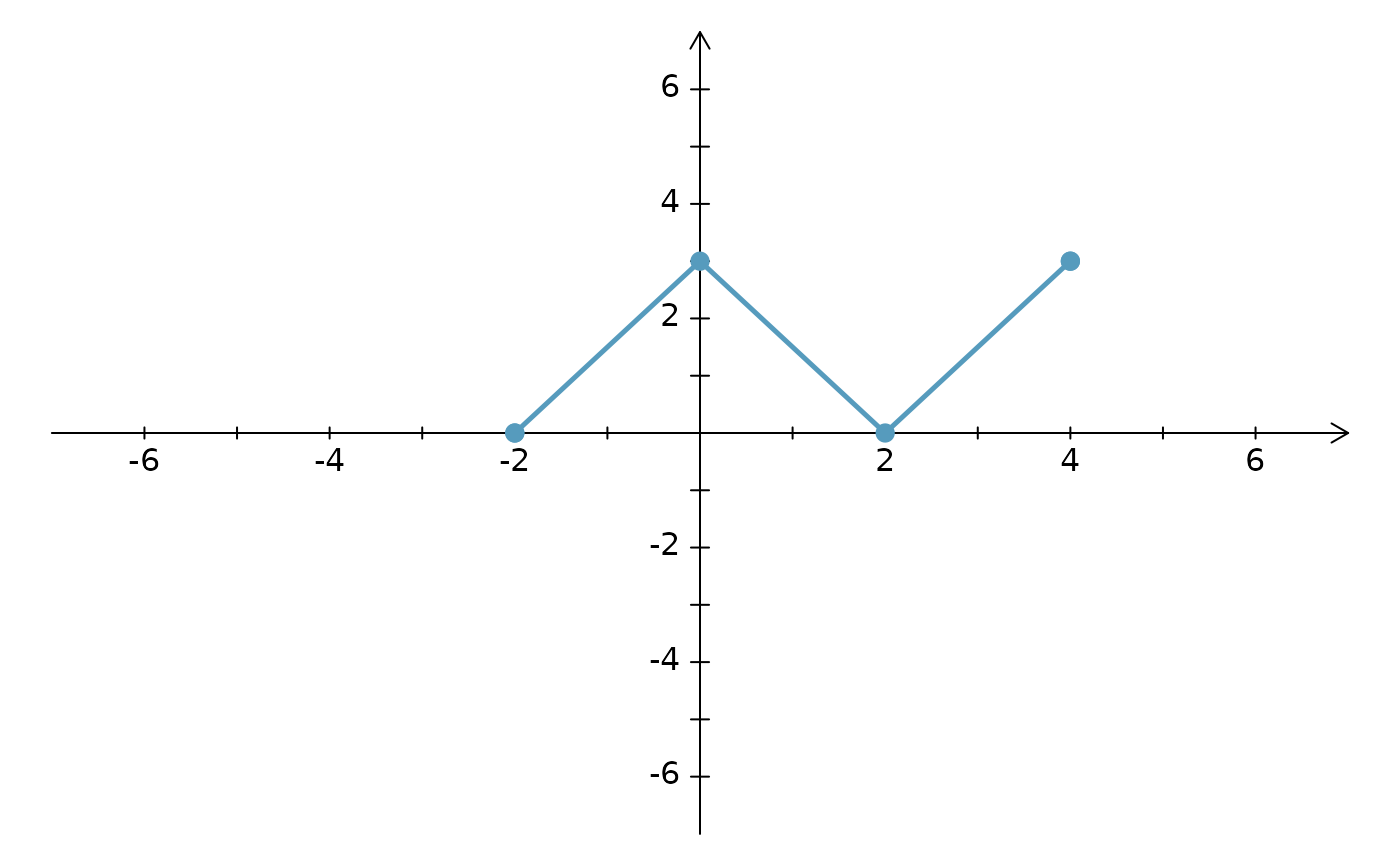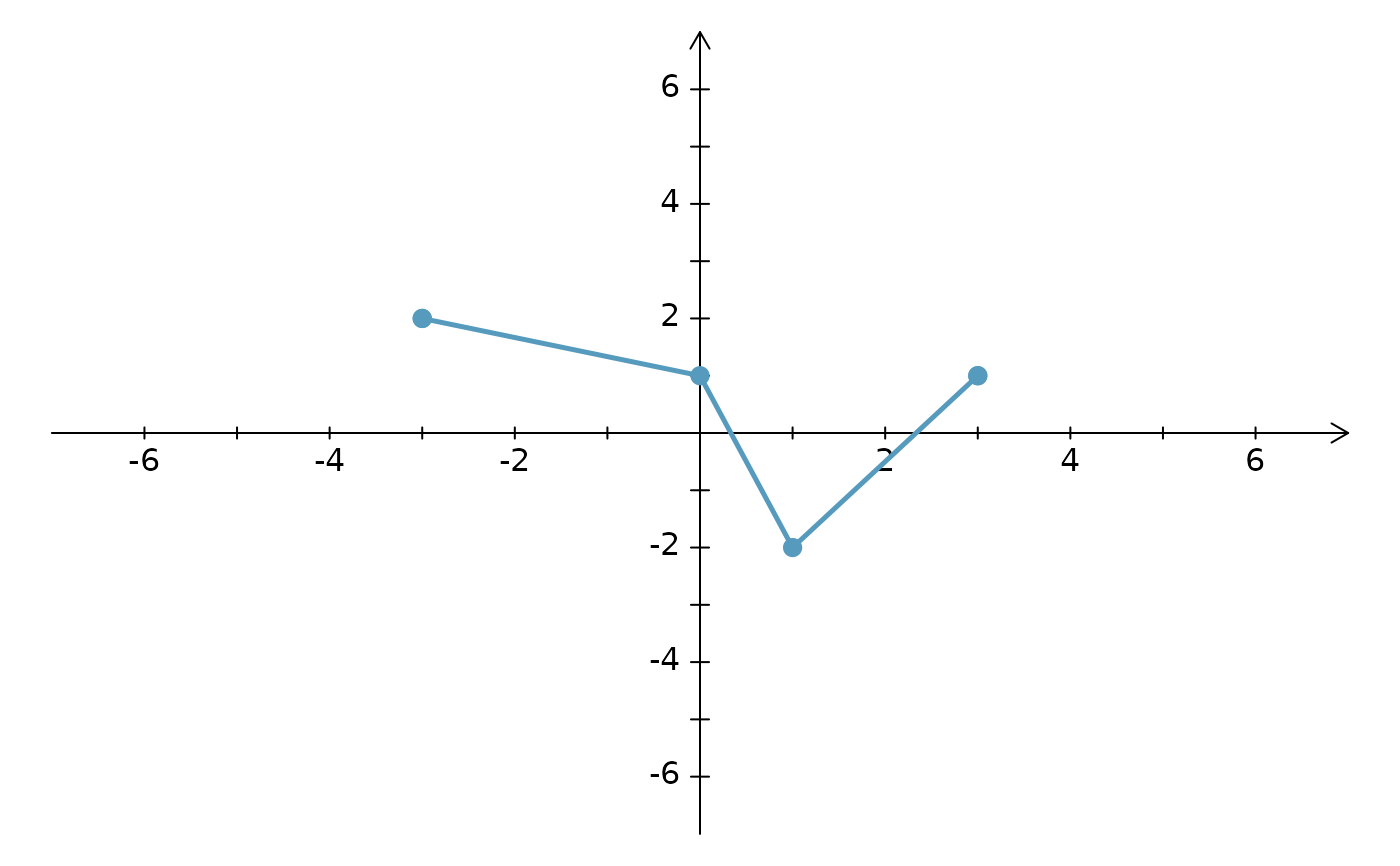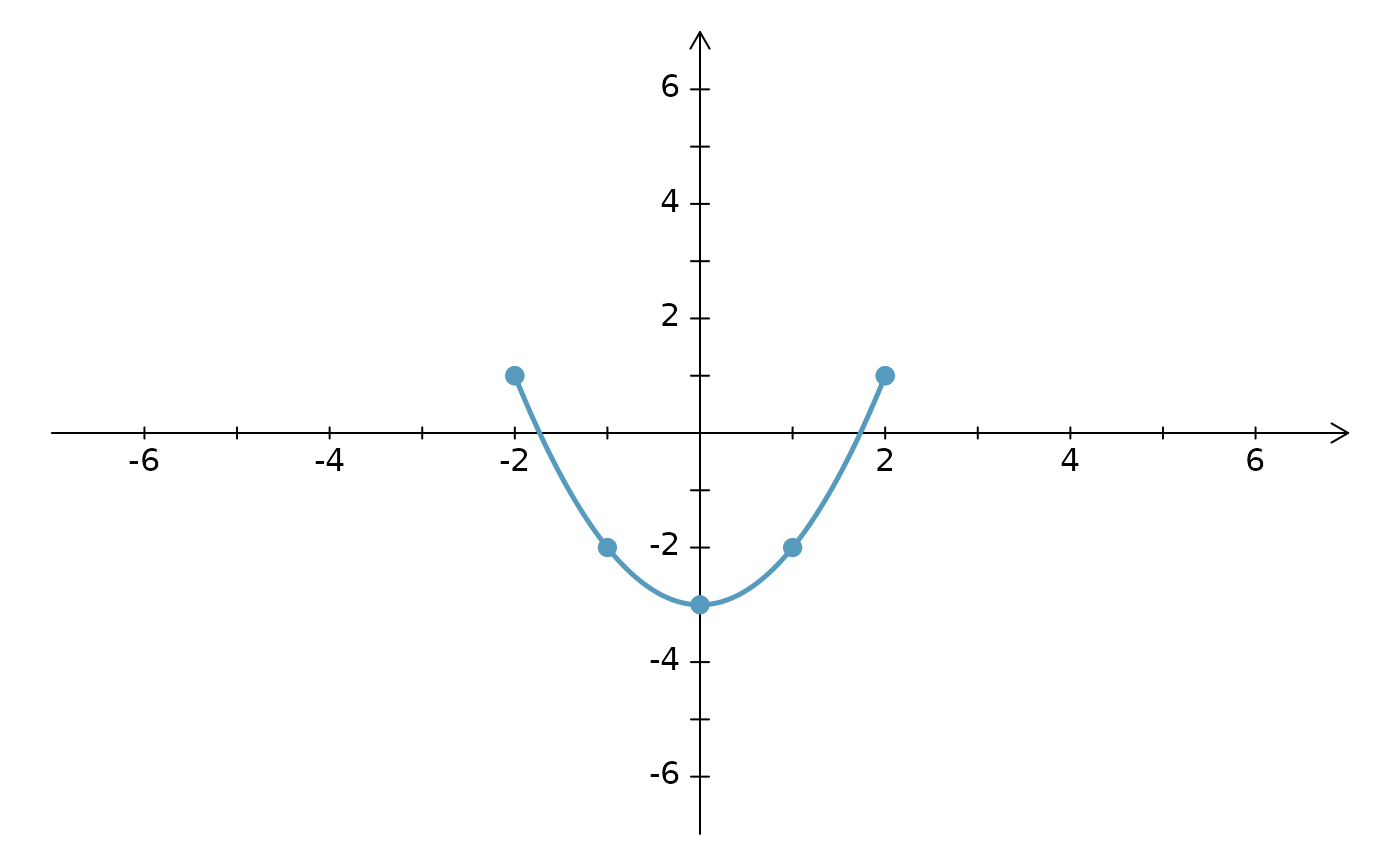Similar to lines, this function will include endpoints that are solid points, open points, or arrows (mix-and-match ready).

## Usage

ArrowLines(
x,
y,
lty = 1,
lwd = 2.5,
col = 1,
length = 0.1,
af = 3,
cex.pch = 1.2,
ends = c("a", "a"),
...
)

## Arguments

x

A vector of the x-coordinates of the line to be drawn.

y

A vector of the y-coordinates of the line to be drawn. This vector should have the same length as that of x.

lty

The line type.

lwd

The line width.

col

The line and endpoint color.

length

If an end point is an arrow, then this specifies the sizing of the arrow. See the length argument in the arrows help file for additional details.

af

A tuning parameter for creating the arrow. Usually the default (3) will work. If no arrow is shown, make this value larger. If the arrow appears to extend off of the line, then specify a smaller value.

cex.pch

Plotting character size (if open or closed point at the end).

ends

A character vector of length 2, where the first value corresponds to the start of the line and the second to the end of the line. A value of "a" corresponds to an arrow being shown, "o" to an open circle, and "c" for a closed point.

...

All additional arguments are passed to the lines function.

lsegments, dlsegments, CCP

David Diez

## Examples


CCP(xlim = c(-6, 6), ylim = c(-6, 6), ticklabs = 2)
x <- c(-2, 0, 2, 4)
y <- c(0, 3, 0, 3)
ArrowLines(x, y, col = COL, ends = c("c", "c"))
points(x, y, col = COL, pch = 19, cex = 1.2)CCP(xlim = c(-6, 6), ylim = c(-6, 6), ticklabs = 2)
x <- c(-3, 0, 1, 3)
y <- c(2, 1, -2, 1)
ArrowLines(x, y, col = COL, ends = c("c", "c"))
points(x, y, col = COL, pch = 19, cex = 1.2)CCP(xlim = c(-6, 6), ylim = c(-6, 6), ticklabs = 2)
x <- seq(-2, 2, 0.01)
y <- x^2 - 3
ArrowLines(x, y, col = COL, ends = c("c", "c"))
x <- seq(-2, 2, 1)
y <- x^2 - 3
points(x, y, col = COL, pch = 19, cex = 1.2)